Monday, December 17, 2018

Data Science in Power BI: Python

Today, we're going to talk about Python in Power BI.  If you haven't read the earlier posts in this series, Introduction, Getting Started with R Scripts, Clustering, Time Series Decomposition, Forecasting, Correlations, Custom R Visuals and R Scripts in Query Editor, the may provide some useful context.  You can find the files from this post in our GitHub Repository.  Let's move on to the core of this post, Python.

In the previous two posts, we've shown how to implement custom visuals and queries using R.  In this post, we'll use the new Python functionality to do the same thing.  Since the Python functionality is still in Preview at the time of writing, we need to enable it in Power BI.Options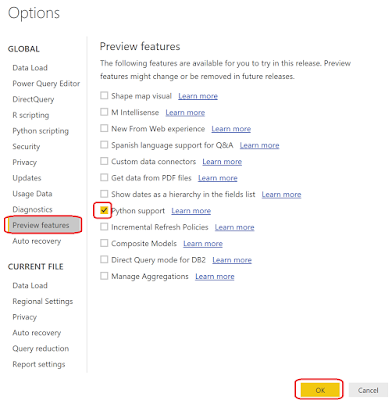Python Support
We can enable this feature by selecting "File" -> "Options and Settings" -> "Options". Then, select "Preview Features" -> "Python Support" -> "OK". We also need to restart Power BI after enabling this feature. After restarting Power BI, we can navigate back to "Options" to see a new tab, "Python Scripting".Python Scripting
In this tab, we need to define a Python directory and an IDE, just like we did with R. We'll be using Anaconda as our Home Directory and VSCode as our IDE.

For the first part of this post, we'll recreate our linear regression model from the Custom R Visuals post. You can download the PBIX here. For reference, here's the R script that we will be recreating in Python.

<CODE START>

`dataset` = read.csv('C:/Users/Brad/REditorWrapper_36d191ca-4dae-4dab-a68c- d15e6b37d3ed/input_df_fe354708-4578-4d89-9272-faf3096df75b.csv', check.names = FALSE, encoding = "UTF-8", blank.lines.skip = FALSE);

# Original Script. Please update your script content here and once completed copy below section back to the original editing window #

dataset[is.na(dataset)] <- 0
reg <- lm(`Revenue Current Month (Log)` ~ . - `Name` , data = dataset )
plot(x = reg\$fitted.values, y = reg\$residuals, xlab = "Fitted Values", ylab = "Residuals",
main = paste( "Predicting Revenue Current Month (Log): R-Squared = ",
round(summary(reg)\$r.squared, digits = 3), sep="")) abline(h = 0)

<CODE END>

First, we need to create a Python visual. It's just easy as it was with R.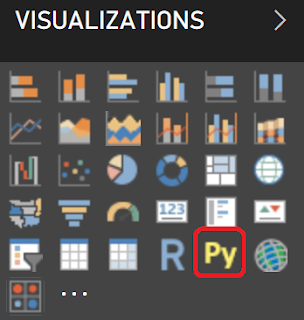Python Visual
We simply need to select the "Py" visual from the visualizations pane.Empty Python Visual
Then, we get an empty Python visual. Let's recreate our chart by dragging on the same fields we used in the R visual.Values
Now, we can open the Python IDE to begin writing our code.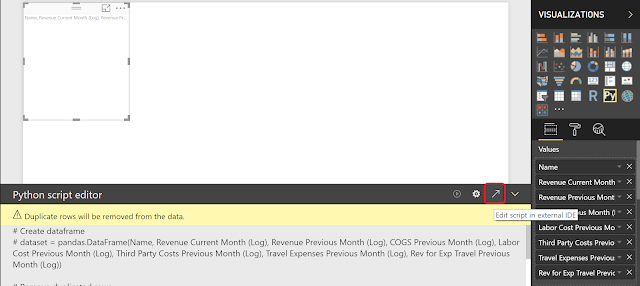Open External IDEBase Script in VSCode
We won't be explaining the intricacies of VSCode in this post. Just think of it an easy way to test our Python code. Let's start by looking at our dataset. Just like in R, it's stored in the "dataset" variable. We can look at this by using the print() and head() functions as follows:

<CODE START>

<CODE END>

<OUTPUT START>

Name                             Revenue Current Month (Log) \
0    NaN                               10.441484
1    ABC Helicopter            11.718719
2    ABISCAS LTD             8.812843
3    Austin Music Club        9.635608
4    BINI Pharmaceutical    12.056482

Revenue Previous Month (Log)   COGS Previous Month (Log) \
0    10.155070                                     9.678040
1    NaN                                              NaN
2    9.554781                                       9.877554
3    9.712569                                       9.885533
4    11.900634                                     12.165114

Labor Cost Previous Month (Log)    Third Party Costs Previous Month (Log) \
0    9.678040                                            NaN
1    NaN                                                    NaN
2    NaN                                                    9.877554
3    9.885533                                            NaN
4   11.578833                                           10.181119

Travel Expenses Previous Month (Log) \
0     NaN
1     NaN
2     NaN
3     NaN
4     10.288086

Rev for Exp Travel Previous Month (Log)
0     NaN
1     NaN
2     NaN
3     NaN
4    10.288086

<OUTPUT END>

Now that we've seen our data, it's a relatively simple task to convert the R script to a Python script. There are a few major differences. First, Python is a general purpose programming language, whereas R is a statistical programming language. This means that some of the functionality provided in Base R requires additional libraries in Python. Pandas is a good library for data manipulation, but is already included by default in Power BI. Scikit-learn (also known as sklearn) is a good library for build predictive models. Finally, Seaborn and Matplotlib are good libraries for creating data visualizations.

In addition, there are some scenarios where Python is a bit more verbose than R, resulting in additional coding to achieve the same result. For instance, fitting a regression line to our data using the sklearn.linear_model.LinearRegression().fit() function required much more coding than the corresponding lm() function in R. Of course, there are plenty of situations where the opposite is true and R becomes the more verbose language.

Let's take a look at the Python code and the resulting visualization.

<CODE START>

from sklearn import linear_model as lm
from sklearn import metrics as m
import seaborn as sns
from matplotlib import pyplot as plt

dataset.fillna( 0, inplace = True )
X = dataset.drop(['Name', 'Revenue Current Month (Log)'], axis = 1)
y = dataset['Revenue Current Month (Log)']
reg = lm.LinearRegression().fit(X, y)

sns.scatterplot(x = reg.predict(X), y = reg.predict(X) - y)
plt.axhline(0)
plt.title('Predicting Revenue Current Month (Log): R-Squared = ' + str( round( m.r2_score( y,
reg.predict(X) ), 3 ) ) )
plt.xlabel('Fitted Values')
plt.ylabel('Residuals')

plt.show()

<CODE END>Residuals vs. Fitted
We see a very similar chart to the one we created in the Custom R Visuals post.

For the second part of this post, we'll receate the custom ETL logic from the R Scripts in Query Editor post. You can download the PBIX here.

Just as with the R script, there is no way to automatically pull the data from the Query Editor into a Python IDE. Instead, we need to write the data out to a csv and upload it into our favorite IDE. Fortunately, Pandas has a very easy function for this, to_csv().

<CODE START>

dataset.to_csv(<path>)

<CODE END>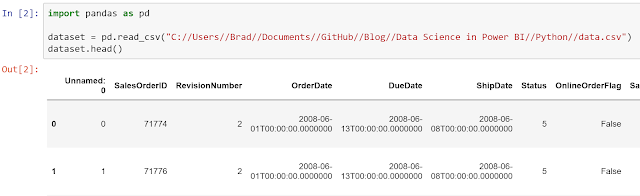Load CSV into Jupyter
When we have a choice, we always go with Jupyter as our Python IDE. It's a notebook, which allows us to see our code and results in an format that is easy to view and export. Next, we can create the "TaxPerc" and "FreightPerc" columns to be used in our dataset.

<CODE START>

tax = dataset[["SalesOrderID", "TaxAmt", "TotalDue"]]
taxperc = tax["TaxAmt"] / tax["TotalDue"]
tax = tax.assign(TaxPerc = taxperc)

freight = dataset[["SalesOrderID", "Freight", "TotalDue"]]
freightperc = freight["Freight"] / freight["TotalDue"]
freight = freight.assign(FreightPerc = freightperc)

<CODE END>Creating New Columns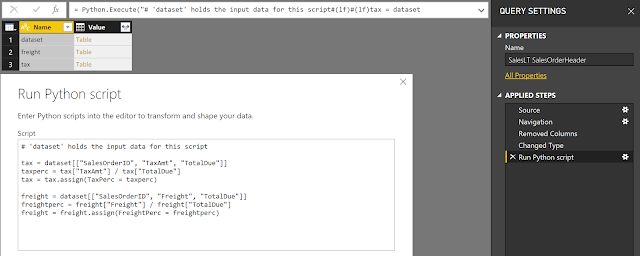Creating New Columns (Query Editor)
One of our personal frustrations with Python is how unintuitive it is to add new columns to an existing Pandas dataframe. Depending on the structure, there seem to be different ways to add columns. For instance, 'tax["TaxPerc"] =', 'tax.loc["TaxPerc"] =' and 'tax = tax.assign(TaxPerc = )' all seem to work sometimes, but not every time. Perhaps someone in the comments can shed some light on why this is. Alas, it's not overly difficult once you find the method that works.

Let's move on to calculating Total Sales per Customer.Total Sales by Customer
<CODE START>

totalsales = pandas.DataFrame( dataset.groupby("CustomerID")["TotalDue"].sum() )
custid = totalsales.index

totalsales = totalsales.assign(CustomerID = custid)

<CODE END>

The code to create a summarization using Pandas is actually quite simple. It's simply "dataset.groupby(<column>).<function>". However, we found an interesting interaction while using this in the Query Editor.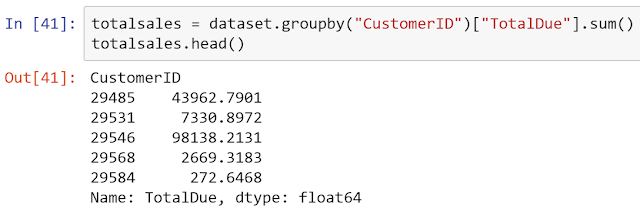Total Sales Series
The line mentioned above actually outputs a Series, not a Pandas DataFrame. This is similar to how the R code outputs a Tibble, instead of an R DataFrame. While the Query Editor was able to read Tibbles from R, it is not able to read Series from Python. So, we needed to cast this to a Pandas Dataframe using the "pandas.DataFrame()" function.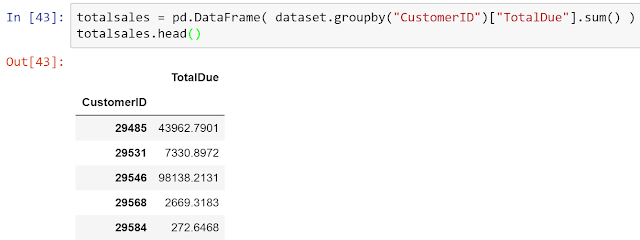Total Sales Dataframe
After this, we found another interesting interaction.  While this may look like two columns in a Python IDE, this is actually a one-column Pandas Dataframe with an Index called "CustomerID". When the Query Editor reads this Dataframe, it throws away the index, leaving us with only the single column.Total Sales by Customer (No CustomerID)
So, we actually had to manually add the "CustomerID" back to the Dataframe using the "assign()" function. It's important to note that the Python functionality is still in preview. Hopefully, they will change this in the future to bring the index into the Query Editor as an additional column.

Finally, let's move on to joining two tables together using Python.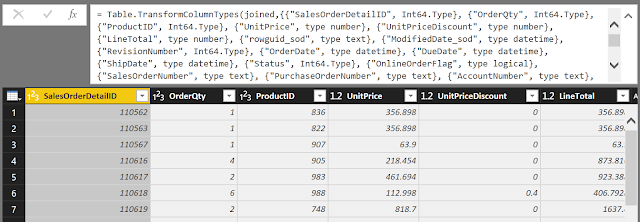Joined
<CODE START>

joined = sod.set_index("SalesOrderID").join(soh.set_index("SalesOrderID"), how = "inner",
lsuffix = "_sod", rsuffix = "_soh")

<CODE END>

We utilized the "join()" function from the Pandas library to accomplish this as well. We prefer to manually define our join keys using the "set_index()" function. As you can see, this isn't much more complex than the R code.

Hopefully, this post empowers you to leverage your existing Python skills in Power BI to unleash a whole new realm of potential. There's so much that can be done from the visualization and data transformation perspectives. Stay tuned for the next post where we'll incorporate one of our favorite tools into Power BI, Azure Machine Learning Studio. Thanks for reading. We hope you found this informative.